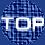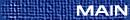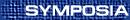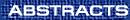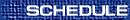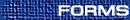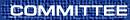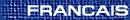Graph Theory / Théorie des graphes(Brian Alspach and Denis Hanson, Organizers) ANTHONY BONATO, Wilfred Laurier University, Waterloo, Ontario  N2L 3C5 New vertex partitions properties of graphs and digraphs A graph G has the pigeonhole property, written (P), if whenever the vertex set is partitioned into two parts, then the subgraph induced by one of the parts is isomorphic to G. In 1996, Peter Cameron proved the surprising result that the only countable graphs with (P) are the trivial graph, the countably infinite complete graph and its complement, and the countably infinite random graph. Last summer, at a conference in honour of Roland Fraïssé, Cameron posed the following problem: which countable graphs G have the property that whenever the vertex set is partitioned into three parts, the subgraph induced by the union of some two of the parts is isomorphic to G? This new vertex partition property is called P(3,2). In this talk, we answer Cameron's problem and present a classification of the countable graphs with P(3,2). A classification of the countable digraphs with P(3,2) will also be presented. This is joint work with Peter Cameron, Dejan Deli\'c, and Stéphan Thomassé. DONOVAN HARE, Okanagan University College, British Columbia Color critical subgraphs of color critical graphs In 1973, J. Nesetril and V. Rödl asked whether, for k ³ 4, a large k-critical graph necessarily contains a large (k-1)-subgraph. The statement is true for k=4 (see references in Problem 5.6 of B. Toft & T. Jensen's book Graph Coloring Problems). In this talk, I will describe a construction for k=5,6, that shows there exists arbitrarily large k-critical graphs on n vertices all of whose (k-1)-critical subgraphs have O(logn) vertices. JING HUANG, University of Victoria, Victoria, British Columbia Convex-round and concave-round graphs Convex-round (concave-round) graphs are those graphs whose vertices can be circularly enumerated so that the (closed) neighbourhood of each vertex forms an interval in the enumeration. These two classes transform into each other by taking complementary graphs. The class of concave-round graphs properly contains the class of proper circular arc graphs and is properly contained in the class of general circular arc graphs. We show that both convex-round and concave-round graphs have nice structural properties and that they can be recognized in O(n+m) time (here n denotes the number of vertices and m the number of edges of the graph in question). We show that the chromatic number of a graph which is convex-round (concave-round) can be found in time O(n+m) (O(n2)). We describe optimal O(n+m) time algorithms for finding a maximum clique, a maximum matching, and a hamiltonian cycle (if one exists), for the class of convex-round graphs. Finally, we show that all convex-round graphs are circular perfect. PETR LISONEK, Simon Fraser University, Burnaby, British Columbia  V5A 1S6 Geometric representations of graphs We will discuss several results on embedding graphs in Euclidean spaces. Applications include constructions of large few-distance sets in Euclidean spaces and graph-theoretic aspects of the Borsuk conjecture. JIM LIU, Lethbridge, Lethbridge, Alberta  T1K 3M4 The total chromatic numbers of joins of sparse graphs In this talk, we investigate the total colorings of the join graphs G1 + G2, where G1 and G2 are graphs with maximum degree at most two. We prove that (1)  when both G1 and G2 are bipartite graphs with maximum degree at most two, then G is Type 1 if and only if G is not isomorphic to Kn,n (n = 1,2,¼) or to K4, and (2)  Cm + Cn is Type 2 if and only if m = n and n is odd. BILL MARTIN, University of Winnipeg/Worcester Polytechnic Institute Bounds on error-correcting codes via the Terwilliger algebra of the n-cube A fundamental problem in the theory of error-correcting digital codes is to determine the asymptotic tradeoff between robustness and message expansion in block codes. The Varshamov-Gilbert bound guarantees, via the probabilistic method, that good codes exist as the length goes to infinity while the linear programming bound of Delsarte is the best known technique for showing that codes do not exist. A recent result of A. Samorodnitsky shows that these two quantities diverge. Thus new constructions are called for and new general techniques for establishing non-existence are needed. In this talk, we restrict attention to the binary case. The Delsarte bound is based on the structure of the adjacency algebra of the n-cube. The Terwilliger algebra is a non-commutative extension of the adjacency algebra which contains more refined information. We propose this algebra as a mode of investigation for improved upper bounds on the efficiency of binary codes. Let Aj denote the adjacency matrix of the distance-i graph of the n-cube and let Ei* denote the diagonal matrix having a one in position (u,u) if u is a binary tuple of Hamming weight i and zeros elsewhere. The matrices Ei* Aj Ek* (omitting copies of the all-zero matrix) give a basis for the Terwilliger algebra. For a code C with characteristic vector c, the statistics cTEi* Aj Ek* c form the coefficients of the biweight enumerator of C which was introduced by MacWilliams, et al. in 1972 and has been studied in relation to linear codes. We show how the Terwilliger algebra sheds new light on this enumerator and how this can be used to obtain some improved bounds on the efficiency of binary codes. The tools we use include graph theory and linear algebra. JOY MORRIS, University of Lethbridge, Lethbridge, Alberta  T1K 3M4 Normal circulant graphs A circulant graph is a Cayley graph X(n;S) on the cyclic group of order n, where S is an inverse-closed subset of Zn that does not contain 0. This graph consists of n vertices labeled 0,¼, n-1, where the vertex labeled i is connected by an edge to the vertex labeled j precisely if i-j mod n is in S. The automorphism group of this graph, consisting of all graph isomorphisms from the graph to itself, is denoted by Aut(X). It is clear that the mapping taking i ® i+j for all i and for any fixed j is an automorphism of this graph. Call this automorphism aj. A normal circulant graph is one for which the cyclic automorphism subgroup consisting of {aj:0 £ j £ n-1} is normal in the full automorphism group. The normal circulant graphs that can also be represented as Cayley graphs on some non-cyclic group of order n are characterised. This result is compared with other results on graphs that can be represented as Cayley graphs on distinct groups of order n. This is based on joint work with Dr. Dragan Marusic of the University of Ljubljana. RICHARD NOWAKOWSKI, Dalhousie University, Halifax, Nova Scotia  B3H 3J5 Well-covered (weightings of) graphs A weighting f: V(G)® R of a graph G is well-covered if the sum of the weights is the same for each maximal independent set of G. A graph is well-covered if the all-ones vector is a well-covered weighting. Caro et al. show that well-covered weightings form a vector space. We follow up the problems of: determining the dimension of this space for families of graphs; determining the non-zero weighted vertices in a basis vector; and indicate what this implies for the problem of characterizing well-covered graphs in general. BRUCE RICHTER, University of Waterloo, Waterloo, Ontario  N2L 3G1 3-connected subsets of the sphere embed uniquely In this joint work with Carsten Thomassen, we prove that if K is a 3-connected, locally connected subset of the sphere S2 and if f: K® S2 is any embedding of K in S2, then there is a homeomorphism h: S2® S2 such that h restricted to K is f. This generalizes the well-known fact that 3-connected graphs have unique embeddings in the sphere. A particular application is that if G is a connected, locally finite graph, then the Freudenthal compactification of G (which adds one point to G for every end of G) has a unique embedding in the sphere. KAREN SEYFFARTH, University of Calgary, Calgary, Alberta  T2N 1N4 Graph products with small cycle double covers A cycle double cover of a graph G is a collection of cycles, C, such that every edge of G lies in precisely two cycles of C. The Small Cycle Double Cover (SCDC) Conjecture, proposed by J. A. Bondy, asserts that every simple bridgeless graph on n vertices has a cycle double cover with at most n-1 cycles, and is a strengthening of the well-known Cycle Double Cover (CDC) Conjecture. Both the CDC Conjecture and the SCDC Conjecture have been verified for various classes of graphs, but remain open in general. The graphs for which that SCDC Conjecture has been verified (including simple triangulations of surfaces, 4-connected planar graphs, and line graphs of numerous types of graphs) all have well defined structural properties that play an important role. The structure that is inherent in graph products makes such graphs ideal special cases for which to verify the SCDC Conjecture. There are various graph products that can be considered, and in this talk I will describe some results and techniques for proving the SCDC Conjecture for certain graph products. This is joint work with R. J. Nowakowski. CLAUDE TARDIF, University of Regina, Regina Saskatchewan  S4S 0A2 A fractional version of Hedetniemi's conjecture Hedetniemi's conjecture states that the chromatic number of a product of two graphs is equal to the minimum of the chromatic numbers of the factors. The inherent difficulty lies in finding lower bounds for the chromatic number of a product of two graphs. In fact, it is not yet known if there exists a number M such that the chromatic number of the product of any two M-chromatic graphs is at least 5. In this talk, we show that the chromatic number of a product of two graphs is at least half the minimum of the fractional chromatic numbers of the factors. ROGER YU, University College of the Cariboo Matching extension problem Let G be a graph. If each k-matching of G can be extended to a perfect matching, then G is called k-extendable (edge version of extension). If after deleting any n vertices the remaining subgraph of G has a perfect matching, then G is called n-factor-critical (vertex version of extension). We will survey the recent progress on the matching extension problem and also discuss the other extensions and the relationship among them.